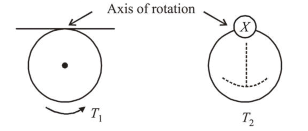# A ring is hung on a nail.Question:

A ring is hung on a nail. It can oscillate, without slipping or sliding (i) in its plane with a time period $T_{1}$ and, (ii) back and forth in a direction perpendicular to its plane, with a

period $T_{2} .$ The ratio $\frac{T_{1}}{T_{2}}$ will be :

1. $\frac{2}{\sqrt{3}}$

2. $\frac{2}{3}$

3. $\frac{3}{\sqrt{2}}$

4. $\frac{\sqrt{2}}{3}$

Correct Option: 1

Solution:

(1) Let $I_{1}$ and $I_{2}$ be the moment of inertia in first and second case respectively.

$I_{1}=2 M R^{2}$

$I_{2}=M R^{2}+\frac{M R^{2}}{2}=\frac{3}{2} M R^{2}$Time period, $T=2 \pi \sqrt{\frac{I}{m g d}}$

$T \propto I$

$\therefore \frac{T_{1}}{T_{2}}=\sqrt{\frac{I_{1}}{I_{2}}}=\sqrt{\frac{2 M R^{2}}{\frac{3}{2} M R^{2}}}=\frac{2}{\sqrt{3}}$